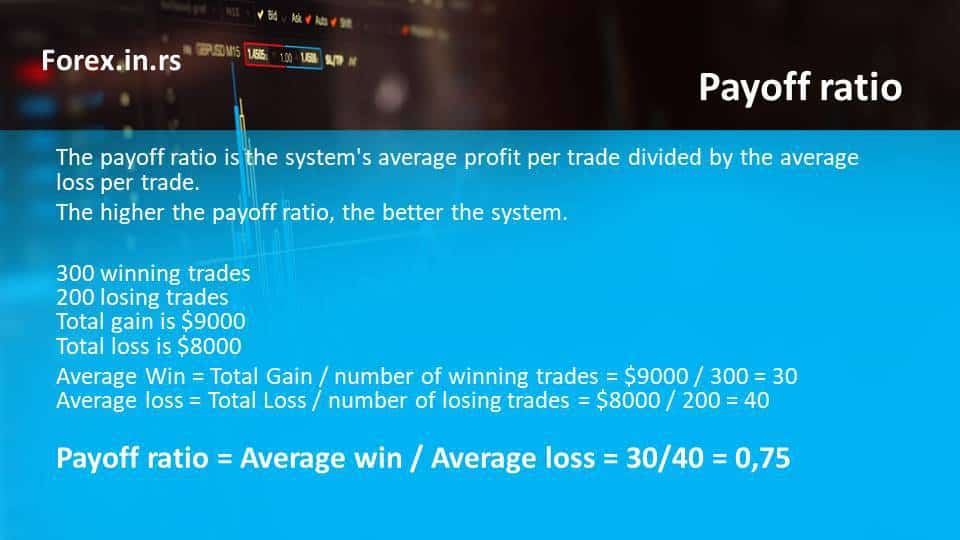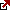# Payoff Ratio

The payoff ratio is a key metric used to measure the success of a portfolio and is an essential factor in determining the overall profitability of investments. The payoff or profit/loss ratio is calculated by dividing the average profit per trade by the average loss per trade. But it’s a measure of how much you make on winning trades versus how much you lose on losing trades. A higher payoff ratio indicates that more profitable trades are being made, while a lower payoff ratio suggests more losses than wins in the portfolio.

The payoff ratio is an essential measure in portfolio management in the financial industry.

The payoff or profit/loss ratio is the portfolio’s average profit per trade divided by the average loss per trade. Simply put, the payoff ratio is the ratio between the size of the win and the loss’s size. If we have higher values – the portfolio performance is better.

The payoff ratio explanation video is below:

This is necessary when discussing money management (visit our article about money management and expert advisors). The payoff ratio is not the same as the Sharpe ratio (the Sharpe ratio is a measure of excess portfolio return over the risk-free rate relative to its standard deviation.)

The importance of understanding the payoff ratio cannot be overstated. It provides investors with valuable insight into their portfolio performance and can significantly impact overall profits. Knowing your payoff ratio helps identify potential areas for improvement and gives you an idea of what strategies may be more effective going forward. Additionally, it can provide insight into which trades have been most successful for your portfolio and which methods to avoid to maximize future profits.

Understanding payoff ratios also allows investors to compare their portfolios against those trading similar assets or strategies. This can enable better decision-making when choosing investments or adjusting existing ones and help them spot trends or patterns they may not have noticed before. Knowing what works best for other investors may help inform one’s own decisions, ultimately leading to improved returns in the long run.

In addition to its utility as an analytical tool, there are also several other benefits associated with using payoff ratios when analyzing portfolios:

• The pay-off ratio metric allows you to assess risk across different positions accurately;
• The pay-off ratio metric will enable you to quickly identify which positions should be adjusted based on recent market movements;
• The pay-off ratio metric provides investors with an easy-to-understand metric for measuring performance;
• The pay-off ratio metric helps you determine whether positions are underperforming or outperforming expectations;
• The pay-off ratio metric enables investors to track their progress over time and adjust their strategy accordingly.

## How to calculate the payoff ratio?

Average win = Total Gain/number of winning trades

Average loss = Total Loss/number of losing trades

Payoff ratio = Average win / Average loss = (Total Gain/number of winning trades ) / (Total Loss/number of losing trades)

## Example of how we can calculate the payoff ratio for a system or portfolio:

Our system or portfolio has the following:

The total gain is \$9000
The total loss is \$8000

Then expected payoff forex ratio will be :

Average Win = Total Gain / number of winning trades = \$9000 / 300 = 30
Average loss = Total Loss / number of losing trades = \$8000 / 200 = 40
Pay off ratio = Average win / Average loss = 30/40 = 0,75What is a good payoff ratio? The payoff ratio above 0,8 is excellent.

About payoff ratio formula and other formulas
We use the Literature payoff ratio when we want to calculate the winning rate or win rate.

Win rate = Profit factor / (profit factor + payoff ratio)

A very similar term is Expected Payoff where

Expected Payoff = Total Net Profit / Total Number of Trades.

## What payoff ratio can tell us about our portfolio?

It can tell us only about the risk-reward strategy, but it can not give an excellent explanation of our portfolio profitability.

We need to calculate average profitability per trade (APPT) or math language expectations except for this value.

Average profitability per trade = (Probability of Win * Average Win) – (Probability of Loss * Average Loss).

So let us calculate the Average profitability per trade for our case :

Three hundred winning trades mean that from 500 trades, 300 are winning. Probability of Win = 300/500 = 60%
Two hundred losing trades mean that from 500 trades, 200 are losing. Probability of loss = 200/500 = 40%
The total gain is \$9000
The total loss is \$8000
Average Win = Total Gain / number of winning trades = \$9000 / 300 = 30
Average loss = Total Loss / number of losing trades = \$8000 / 200 = 40

Average profitability per trade = (Probability of Win * Average Win) – (Probability of Loss * Average Loss) = 0.6*30 – 0.4*40 = \$18 – \$16 = \$2

## Payoff ratio vs. Profit factor

The profit factor is a better signal than the payoff ratio. The payoff ratio can be meager, and the system can be profitable. The payoff ratio without a win rate can not be used as a measure.

So if we know that :
The profit factor is the ratio when we divide profit from winning trades by the loss of losers.
The Win rate is the percentage of winning trades based on the number of total trades.
The payoff ratio is the average winning trade divided by the average losing trade.

There is a rule about the Profit factor and payoff ratio :

The profit factor will stay constant if we lower the payoff ratio only if we develop a higher win rate strategy.

Payoff ratio calculator

How to count profit in forex?

In forex, the best way to calculate profit is to use percentages. In that way, you can measure daily, monthly, or yearly percentage gain. For example, the best trading performance is a 20% annual profit gain.

One important thing that we need to separate is one of the most common questions in finance.
Is the payout ratio the same as the payoff ratio? The payout ratio shows the proportion of earnings paid out as dividends to shareholders. The payoff ratio is the portfolio’s average profit per trade divided by the average loss per trade.

## Conclusion

All in all, having a good understanding of pay-off ratios is essential for any investor looking to maximize returns from their portfolio. By giving insights into trade performance and potential areas for improvement, it helps ensure that decisions are made with full knowledge of up-to-date market conditions and allows traders to react quickly when changes occur – ultimately helping them stay ahead of the curve and maximize profits in any given situation.

Fxigor

Igor has been a trader since 2007. Currently, Igor works for several prop trading companies. He is an expert in financial niche, long-term trading, and weekly technical levels. The primary field of Igor's research is the application of machine learning in algorithmic trading. Education: Computer Engineering and Ph.D. in machine learning. Igor regularly publishes trading-related videos on the Fxigor Youtube channel. To contact Igor write on: igor@forex.in.rs

Trade gold and silver. Visit the broker's page and start trading high liquidity spot metals - the most traded instruments in the world.